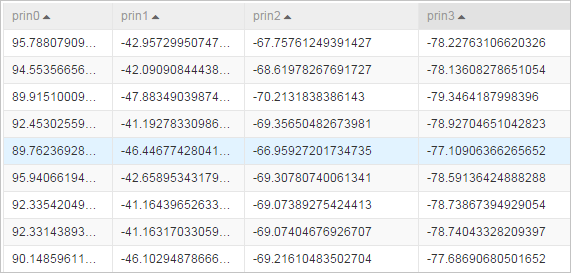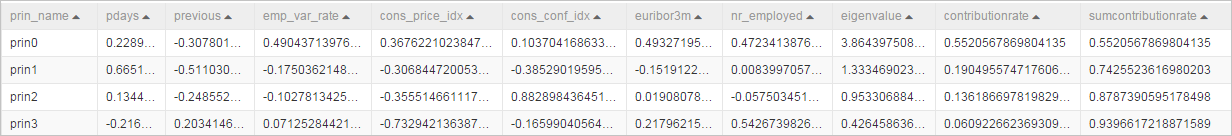Principal component analysis (PCA) is a multivariate statistical method used to explore the internal structures of multiple variables and how they correlate to each other based on a few principal components. You can use PCA to export a few principal components that are unrelated to each other from original variables. These principal components retain as much information about the original variables as possible and are used as new comprehensive metrics.

## Limits

The PCA component supports only data in the dense format. You can use the component for dimensionality and noise reduction.

## Configure the component

You can configure the component by using one of the following methods:
• Use the Machine Learning Platform for AI console
Tab Parameter Description
Fields Setting Feature Columns The columns that are selected from the input table for analysis.
Appended Columns The columns that are appended to the table after dimensionality reduction.
Parameters Setting Data Volume Ratio The information retaining ratio after dimensionality reduction.
Feature Decomposition Mode The method that is used to decompose features. Valid values:
• CORR
• COVAR_SAMP
• COVAR_POP
Data Conversion Method The method that is used to convert data types. Valid values:
• Simple
• Sub-Mean
• Normalization
Tuning Lifecycle The lifecycle of the output table. The value of this parameter must be a positive integer.
Number of Nodes The number of cores. This parameter must be used with the Memory Size per Node parameter. The value of this parameter must be a positive integer. Valid values: [1,9999].
Memory Size per Node Unit: MB. The memory size of each core. The value of this parameter must be a positive integer. Valid values: [1024,64 × 1024].
• Use commands
``````PAI -name PrinCompAnalysis
-project algo_public
-DinputTableName=bank_data
-DeigOutputTableName=pai_temp_2032_17900_2
-DprincompOutputTableName=pai_temp_2032_17900_1
-DselectedColNames=pdays,previous,emp_var_rate,cons_price_idx,cons_conf_idx,euribor3m,nr_employed
-DtransType=Simple
-DcalcuType=CORR
-DcontriRate=0.9;``````
Parameter Required Description Default value
inputTableName Yes The input table that is used for training. N/A
selectedColNames Yes The columns that are selected from the input table for analysis.

Separate multiple columns with commas (,). The columns of the INT or DOUBLE data type are supported.

N/A
eigOutputTableName Yes The output table that contains feature vectors and feature values. N/A
princompOutputTableName Yes The output table after dimensionality and noise reduction of principal components. N/A
transType No The method that is used to transform the original table to a PCA table. Valid values:
• Simple
• Sub-Mean
• Normalization
Simple
calcuType No The method that is used to decompose features of the original table. Valid values:
• CORR
• COVAR_SAMP
• COVAR_POP
CORR
contriRate No The information retaining ratio after dimensionality reduction. Valid values: (0,1). 0.9
remainColumns No The fields that are retained from the original table after dimensionality reduction. N/A
coreNum No The number of cores. This parameter must be used with the memSizePerCore parameter. The value of this parameter must be a positive integer. Valid values: [1,9999]. Determined by the system
memSizePerCore No The memory size of each core. Unit: MB. The value of this parameter must be a positive integer. Valid values: [1024,64 × 1024]. Determined by the system
lifecycle No The lifecycle of the output tables. The value of this parameter must be a positive integer. N/A

## Example

Sample output tables of the PCA component
• Data table after dimensionality reduction• Table that contains feature values and feature vectors